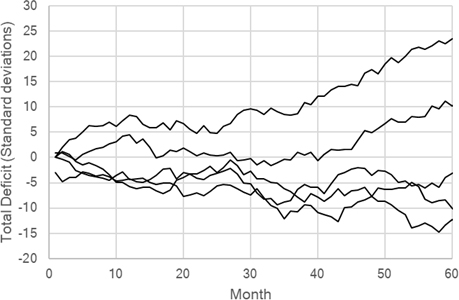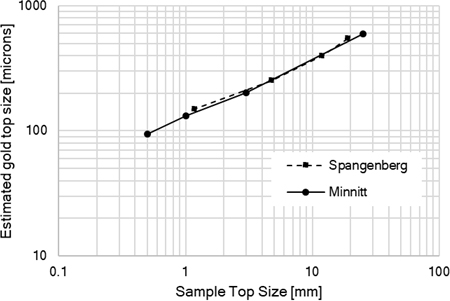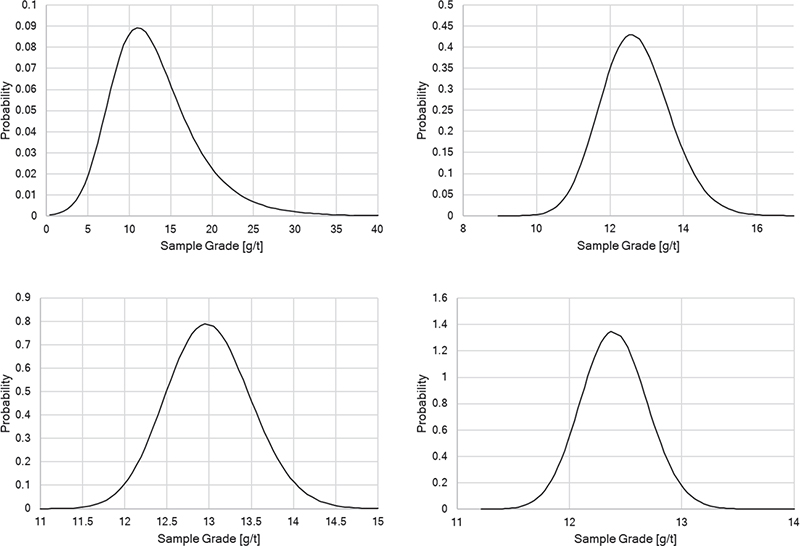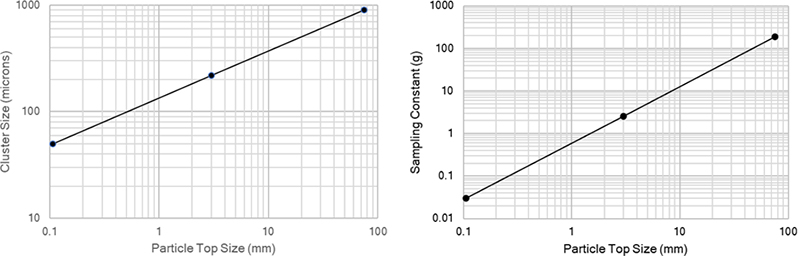# Sampling of gold ores for commercial purposes

Full-Text PDF

#### Geoff Lyman

Materials Sampling & Consulting. [email protected]

Let us take the example of the sampling of a gold ore coming from a small high grade deposit where the ore is to be beneficiated at a third party concentrator. There are two reasons why the ore must be sampled in an accurate manner. First, there must be a good estimate made of the contained gold so that the mine pays royalties to the state correctly. Second, the contract with the concentrator needs to pay the miner fairly for the gold contained in the ore and apply penalties for deleterious elements also contained in the ore as determined from the assays of the incoming ore. In this example, we show the impact of sample precision on the possible cash flows for the concentrator or the miner. It is assumed that the sampling is “correct”, this is, that it is unbiased. The matter of whether the sampling is “representative” hangs on whether the sampling is “fit for purpose” (which is the real meaning of representative sampling) and can be judged by whether or not the economic risks faced by the parties involved are acceptable.

This example is based on an actual mine/concentrator collaboration, except that the grades and ore characteristics have been altered somewhat for reasons of confidentiality.

The ore is taken to be a difficult one containing coarse gold at a mean grade of 30 g/t and showing individual small bulk sample grades up to 180 g/t and down to less that 2 g/t. The distribution of sample grades is heavily skewed and follows an approximate log–normal distribution of grade, as might be expected. The standard deviation of the grades is very close to the mean grade. Production from the mine will be in daily 400 tonne batches which will be sequestered at the mine prior to shipment. Each batch will be sampled and assayed in order to determine if it is high enough grade to be sent to the concentrator. The ore will also be sampled again as received at the concentrator.

The critical question is how precise the daily sampling must be in order to control the risk of under- or over-payment for the ore over a period of time. The uncertainties due to sampling, sample preparation and analysis attached to the assays upon which payments are based are statistically independent and can be positive or negative and may be normally distributed. The assays can be viewed as true metal contents with a random uncertainty added to each one. From the point of view of a single assay upon which payment is made, the uncertainty may be positive or negative leading to an over-payment or under-payment, the magnitude of which is directly related to the variance (or standard deviation) of the uncertainty.

However, taking a longer-term view, it will happen that a run of positive or negative uncertainties can occur which will leave the mine or concentrator with a temporary deficit. If the concentrator is on the losing end of this run, they will be genuinely out of pocket as they will have over-paid the mine. This will have a direct impact on their cash flow as the gold they have paid for will not arrive at the bullion room. If the miner is on the losing end, he will be none the wiser unless his exploration and mine plan is so good that he can detect the fact that fewer ounces of gold have been realised from the mined ore than predicted from the mine plan. Nonetheless, he will be less well-off than he should be and this will impact his cash flow.

It is quite possible to make some simple calculations which show the extent to which the positive or negative runs of assay uncertainties can add up. Figure 1 shows five realisations of how positive or negative uncertainties can occur and add up to a significant value after a series of payments on a monthly basis. The magnitude of the deficit or surplus is measured in standard deviations of the uncertainty. In four out of the five cases, the difference from the true value has reached ten standard deviations after 60 months or 5 years or less.Figure 1. Random accumulation of surplus or deficit on payments in terms of assay standard deviations.

If the little mine ships ore 5 days a week, we can count 20 days as the nominal payment period and the ore shipped will be nominally 8000 tonnes. At an average grade of 30 g/t this is 240,000 g or 7717 ounces. At a value of $1700/oz, this is$13.1 million. Now assume that the standard deviation of the uncertainty at the end of the payment period is 4 %. Then one standard deviation corresponds to $0.524 million, five standard deviations to$2.62 million and ten standard deviations to \$5.24 million.

While these figures are relatively small compared to the overall revenue from the mine, the value is significant and even a deficit of a few standard deviations is enough to cover the cost of a well-designed sampling system for the mine. With advance planning, a sampling system can be put together from second-hand equipment that will be capable of delivering results that might be able to improve on the uncertainty of 4 % relative on the 20 day payment period.

## Achieving accurate sampling of coarse gold ores

There has been much discussion of how to work out a satisfactory sampling protocol for ores containing coarse gold. There has also been debate on exactly what constitutes a “coarse” gold ore. And there has been debate on how to pulverise a gold ore containing “coarse” grains of gold without having the gold smear onto the surface of the grinding equipment with the loss of gold.

Then there is the problem of assaying a sample before or after pulverisation. There are now two methods of dealing with relatively large samples of gold ore that can be submitted for analysis without pulverisation to pass 150 µm or 106 µm.

The first is the Pulverise and Leach (PAL) system that accepts a 1 kg sample of ore up to about 5 mm in size and puts it in an iron pot with grinding balls and an accelerated CN leach solution and tumbles the pot for about one hour. At the end of the tumbling, both the ground solids (now 75 µm or so) and the supernatant solution can be recovered. The solution can be analysed directly and the solids recovered, rinsed, dried, weighed and subjected to fire assay. Multiple 1 kg subsamples of the same ore can be used as determined by the analysis protocol. The advantage of the method is the large sample mass possible and the fact that there can be no loss of gold to smearing as such gold will be dissolved.

The second method is the new Photon Assay procedure brought to a commercial readiness by the CSIRO in Australia and now being rolled out in analytical labs and dedicated corporate facilities across the world. In simple terms, the method uses samples up to 500 g in mass contained in a jar and the jar is irradiated by 8–10 MeV x-rays which are highly penetrating of the ore and excite the gold nuclei which then decay with the emission of 279 keV gamma-rays, which are also highly penetrating. Multiple 500 g samples crushed only to <~2 mm can be used for an ore. The method is non-destructive. Current data show the method to be more accurate than any other methods for samples above about 1 g/t. The approximate standard deviation of an assay at 1 g/t is 2.5 % relative and reduces as the sample grade increases, as indicated by available literature. The method has also been extended to Ag, Cu and moisture analysis.

Both methods are relatively cheap as sample preparation is minimised, but the PAL method does require fine assay of the residual solids to ensure that all the gold is captured.

The key to understanding the problems of gold analysis when the gold grains or gold grain clusters are coarse is to recognise that the size distribution of the gold grains/clusters controls the number of gold grains/clusters to be found in a sample of a given mass. The number of grains/clusters of a given size (or equivalent mass) in a sample follows a Poisson distribution and this fact permits calculation of the distribution of grades that will be observed over correctly sampled subsamples of the ore for the ore in the state of comminution at hand. It also permits a simple calculation of the sampling variance for the ore subsamples. It does not matter what the state of comminution the ore is in; it matters only that the size (mass) distribution of the grains be known or can be estimated with reasonable precision. Further, if it is legitimate to assume that the mass distribution of the grains/clusters can be assumed to follow the often-seen Rosin–Rammler (Weibull) distribution, the sampling variance can be written in terms of the 95 % passing size of the grains/clusters, a grain/cluster shape factor and a parameter describing the breadth of the mass distribution.

In the author’s development of statistical sampling theory.1 the sampling variance due to the intrinsic (constitutional) heterogeneity can be written in terms of a sampling constant for the element of interest, KS, as

$${{\sigma _{IH}^2} \over {\bar A_L^2}} = \left[ {{1 \over {{M_S}}} - {1 \over {{M_L}}}} \right]{K_S}$$

(1)

where the mean grade is AL, and the sample mass is MS, ML is the mass of the lot from which the sample is taken and σ2 is the sampling variance due to the element of interest. In a simple case where the gold grain mass distribution is unimodal, the sampling constant can be shown to be

$${K_S} = {{{\rho _{Au}}} \over {{A_L}}}fgd_{95\;Au}^3$$

(2)

where ρAu is the density of the gold, f is a shape factor, g is a size distribution factor having a value not too different from 0.25 and d95 Au is the 95 % passing size (by mass) of the gold grains/clusters. The sampling constant has units of mass. The validity of this formulation of the sampling variance for a gold ore has been tested against the excellent (but very rare) data on gold sampling variance as a function of the top size to which the material was crushed.1–3

The fact that the number of gold grains in a set of gold mass fractions in an ore follow a Poisson distribution can be used to calculate the so-called characteristic function for the sampling distribution of the ore and this function can be inverted to provide the probability density function. This capability is a new tool in sampling theory that can be used to shed light on the impact of gold grain/cluster size on sampling variance and particularly on the skewness of the sampling distribution.

Figure 2 shows the 95 % passing size of gold grains/clusters calculated from the observed variance over 30 nominally identical subsamples at each top size for a ~12 g/t gold ore. It is likely that the gold at the larger top sizes is in the form of substantial clusters and not discrete compact grains.Figure 2. Gold grain/cluster top size estimated for data of Minnitt et al. and Spangenburg from observed sampling variance estimated from individual assays of 30 nominally identical subsamples assayed to extinction.

The observed behaviour, virtually identical for two independent analyses of the same type for a single gold ore, shows a reasonable log–log decrease of estimated top size of the grains/clusters as a function of top size to which the ore was crushed. This permits the calculation of the sampling variance at any intermediate sizes and may permit some extrapolation to larger or smaller sizes. Clearly, what are probably clusters are being broken down with the crushing until at 0.5 mm top size the clusters have been broken into grains.

It is also interesting to compare the sampling probability density functions calculated for the data of Minnit. These are shown in Figure 3. The skewness of the distribution is clear at the 25 mm top size. Note also that the density functions calculated provided an excellent match to the actual distribution of the 30 results at each top size.Figure 3. Sampling probability density functions for the ore at a series of top sizes to which the ore was crushed. 25.0, 3.0, 1.0, 0.5 mm, top to bottom, left to right. Sample mass is 273 g in all cases.

The ore characterisation provided by the method of creating set of nominally identical subsamples of the ore and analysing to extinction to permit calculation of the variance over the subsamples and interpreting the results by the method presented here is far more useful and sensible than attempting to interpret the data according to Gy’s so-called K-α model which has caused difficulties and controversy for many years now.

To sample a gold ore and achieve a result with a controlled overall sampling variance, it is necessary to consider all sources of variance that impact the total sampling and analysis variance. The sampling of a run of mine ore is the most difficult task as the ore grade can vary substantially in the raw ore coming from one or more mining faces. The mine plan and the in situ grade estimation data upon which the mine plan is based is the only source of information at the early stage of mine development. It is better to over-estimate the variability than to be tempted to believe the ore is more homogeneous than it might be. Next it is mandatory to have an estimate of the ore heterogeneity as determine by the sampling constant for the ore at various top sizes to which it might be crushed. The variation of the heterogeneity (as quantified by the sampling constant) with the size to which the ore is crushed must be established by a test similar to the procedure described above. Only then can a sampling system be correctly designed in a way that will stand up to scrutiny under commercial sampling conditions.

## Example

Let us take the case envisaged above and consider the design of a sampling system that will achieve very good results even when the average grade for a lot is lower than the overall average. Note that the sampling constant for the ore is inversely proportional to the ore grade so that low grade or is more heterogeneous than high grade ore. With the objective of considering a somewhat worse case than average, this example will take the average grade to be 10 g/t with a standard deviation of feed to the sampling plant of 15 g/t. The lot mass for sampling is 400 t, which production from one day which is to be classified as ore or waste. The grade variation in the feed to the sampling plant will be taken to be random with the standard deviation of 15 g/t. The analysis will be assumed to be carried out by Photon Assay with a standard deviation of 1.5 % relative (the grade is above 1 g/t). It will be assumed that the ore is fed to the sampling plant over a 2–3 hour period and design will be for 2 hours or primary feed. The 95 % passing size of the feed is 75 mm.

The variance due to the time variation of the feed grade (distributional heterogeneity) is determined by the number of increments taken over the lot by the primary sampler.

$$\sigma _{DH}^2 = {{\sigma _{feed}^2} \over {{N_{inc}}}}$$

(3)

$${M_{pri}} = {N_{inc}}{{Qw} \over {3.6v}}$$

(4)

where Q is the feed rate in tph, w is the aperture in metres (≥3d95feed) and v is the cutter velocity in m/s (max 0.6 m/s). The mass is given in kilograms. The primary increments are crushed to 3 mm and sampled by a secondary sampler and the collected mass of the secondary increments is determined by a similar formula.

To determine the variance due to the IH of the ore at the primary and secondary stage of sampling, Equation (1) is used with appropriate values of the sampling constant.

The optimisation of the sampling protocol is best done by setting up a spreadsheet using the formulae provided herein and then working with the number of primary increments collected and the mass divisions at each stage of sampling. It is never immediately apparent where the controlling variance will appear.

The heterogeneity of the ore is controlled by the grain/cluster sizes in the ore. In what follows it has been assumed that at effective sizes are 900, 220 and 50 µm at top sizes of 75, 3 and 0.106 mm. These are plotted in Figure 4. Also plotted are the sampling constants at the three top sizes.Figure 4. Assumed gold grain/cluster sizes and calculated sampling constants at 0.160, 3 and 75 mm.

The variance budget for the sampling system after optimisation is provided in Table 1.

Table 1. Variance budget for the sampling system after optimisation.

 Component Relative variance Relative standard deviation (%) Primary sampling DH 9.37E-03 9.68 Primary sampling IH 2.5063E-05 0.50 Secondary sampling IH 0.000084 0.92 IH due to splitting of secondary increments 0.001186 3.44 Analysis variance by Photon Analysis 0.00005625 0.75 Total for 400 tonne lot 1.07E-02 10.36 Total for 20 lots per month 2.32

The optimisation indicated that the most critical aspect of the system was due to primary sampling DH. It was necessary to sample at 30 second intervals to bring the variance down. This then dictated the secondary sampling, which involved feeding the primary increments collected in a bin over a 4-hour period. This change from 2 to 4 hours was dictated by the need to collect at least six secondary increments for each primary increment. The mass of primary increments collected was 7500 kg and the mass of secondary increments collected per lot was 30 kg with crushing of primary increments to 3 mm.

The 30 kg of secondary increments was split to 2 kg at which point either four aliquots at 0.5 kg or two aliquots at 1.0 kg could be formed, the first for four replicate Photon Assays with a relative standard deviation of 1.5 % per assay and the latter for duplicate 1 kg screen fire assays with pulverisation to 106 µm. The assay uncertainty for the screen fire assays was estimated to be larger than the Photon Assays, assuming a relative standard deviation for a single fire assay of 4 %.

The results from this sampling example are very good for the monthly average relative standard deviation of 2.32 %. It is clear that in this case, the critical issue is taking a sufficient number of primary increments from the highly variable feed. The IH of the ore manifests itself through the variance component due to splitting the ore at a size of 3 mm. Reduction of the ore past 3 mm is not necessary for Photon Assay and the Photon Assay method eliminates the sample preparation of the ore to nominally passing 106 µm with screen fire assay at 76 µm. The possible losses of gold in the preparation process are eliminated.

## Conclusion

The material presented has explained the issues involved in the sampling for highly variance coarse gold ore based on heterogeneity assumptions that are in line with the heterogeneity found by Minnitt et al. for Witwatersrand ore. The calculations underline the fact that it is not generally possible to guess where the critical point in the sampling system design will occur and the value of having a reasonable estimate of the ore heterogeneity as a function of ore top size. The calculations highlight the value of modelling the sampling constant for the ore as a function of gold grain/cluster top size. Clusters of grains are clearly important to deal with.

## References

1. G.J. Lyman, “Mathematical developments in particulate sampling theory”, P.M. Gy Oration, Ninth World Conference on Sampling and Blending, Beijing, China (May 2019).
2. R.C.A. Minnitt, P.M. Rice and C. Spangenberg, “Part 2: Experimental calibration of sampling parameters K and alpha for Gy’s formula by the sampling tree method”, J. S. Afr. Inst. Min. Metall. 107, 513–518 (2007). https://www.saimm.co.za/Journal/v107n08p513.pdf
3. G.J. Lyman, Theory and Practice of Particulate Sampling Theory – an Engineering Approach. Materials Sampling & Consulting, Brisbane (2019). http://www.materials-sampling-and-consulting.com/textbook### Geoffrey Lyman

Geoffrey Lyman has worked widely in mineral processing research and mathematical modelling for many decades. His current work is in sampling of particulate materials, through his company Materials Sampling & Consulting Pty Ltd, which also provides courses to in-house groups or at Conferences. He has worked on sampling in a wide variety of industrial sectors, i.e. in the food industry, the grain industry and widely in minerals sampling (gold, platinum group elements—concentrators, smelters and autocatalyst recycling—coal, iron ore and base metals). He has many authored leading papers in the statistical theory of sampling over the last five years. He has recently developed a means of calculating the entire probability distribution for the sample analyte content. A major new textbook was published in 2019, in which he takes a final step forward past the sampling theory of Gy.
[email protected]